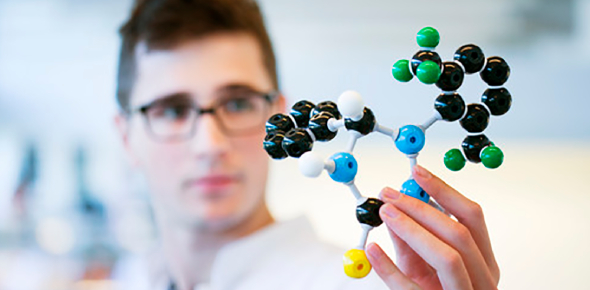# Gases Quiz - Learn Chemistry

25 Questions | Attempts: 21SettingsGas is one of the four fundamental states of matter. A pure gas may be made up of individual atoms, elemental molecules made from one type of atom, or compound molecules made from a variety of atoms. A gas mixture, such as air, contains a variety of pure gases. Improve your Chemistry Knowledge in this Gases Quiz!

• 1.
What will be the volume of solution in dm3 , when 6.02 × 1022 molecules of sugar are present in its 1M solution
• A.

1 dm3

• B.

0.1 dm3

• C.

0.01 dm3

• D.

10 dm3

• 2.
When 2g H2 gas and 16g O2 gas react completely to produce H2O. What is non-limiting reactant
• A.

Hydrogen

• B.

Oxygen

• C.

Water

• D.

Both are used Completely

• 3.
How much oxygen is required to react completely with 54g of Al to form alumina
• A.

24g

• B.

48g

• C.

72g

• D.

96g

• 4.
1 mole of carbon is present in one mole of which of the following species/substance
• A.

CaCO3

• B.

CH3CHO

• C.

CH3COOH

• D.

CaC2

• 5.
The term relative atomic mass is used for
• A.

37 amu Cl

• B.

100 amu Ca

• C.

35.5 amu Cl

• D.

96 amu S

• 6.
What are the ratio among the volumes of gases 4He ,16CH4 and 32O2, if they are taken in equal masses at STP
• A.

1 : 2 : 8

• B.

1: 1: 1

• C.

1 : 8 : 2

• D.

8 : 2 : 1

• 7.
The molecular mass of D2O is
• A.

18.02 amu

• B.

19.04 amu

• C.

20.03 amu

• D.

22.04 amu

• 8.
Total number of carbon atoms are present in 44g of CO2
• A.

0.5 NA

• B.

2NA

• C.

1 NA

• D.

3NA

• 9.
One mole of each of the following compounds is burnt in excess of oxygen. Which compound will produce three moles of carbon dioxide and three moles of steam only
• A.

C3H8

• B.

C3H7CO2H

• C.

C3H7OH

• D.

CH3COOCH3

• 10.
What is volume occupied by 4.4g of N2O at STP
• A.

22400cm3

• B.

2240 cm3

• C.

1120 cm3

• D.

120 cm3

• 11.
11200 cm3 of oxygen gas is collected at STP from Hydrilla plant by photosynthesis. The mass of oxygen gas produced is
• A.

32 g

• B.

16 g

• C.

8 g

• D.

4 g

• 12.
Which of the following has minimum mass
• A.

6.02×1023 molecules of H2

• B.

22.4 dm3 of He

• C.

0.25 mole of Ne

• D.

10g of Ca

• 13.
3×NA number of ionizeable H+ are present in 1 mole of
• A.

H2SO4

• B.

H3BO3

• C.

H3PO4

• D.

CH3COOH

• 14.
What is the mass of CaCO3 which on heating produces 0.25 moles of CO2 gas
• A.

12.5 g

• B.

25 g

• C.

50 g

• D.

100 g

• 15.
The mass of one mole of electron is
• A.

9.1 × 10–31 kg

• B.

1.602 × 10–19 kg

• C.

0.55 mg

• D.

0.55g

• 16.
The number of carbon atoms in 45g of C6H12O6
• A.

6 × NA

• B.

3 × NA

• C.

1.5 × NA

• D.

0.25 × NA

• 17.
N2 (g)  + O2 (g) -------------->   2NO (g)  Volume of nitric oxide gas produced by the following reaction of 7g N2 with excess of oxygen is
• A.

22.4dm3

• B.

5.6 dm3

• C.

11.2 dm3

• D.

2.8 dm3

• 18.
Number of grams of NaOH required for complete neutralization of 1mole of H3PO4 on its complete ionization
• A.

120 g

• B.

100 g

• C.

80 g

• D.

40 g

• 19.
H2O, HF and Na+ have same
• A.

Mass Number

• B.

Number of Electrons

• C.

Number of protons

• D.

Number of neutrons

• 20.
What volume of NH3 is produced on reacting 20cm3 of N2 with 80cm3 of H2
• A.

80 cm3

• B.

40 cm3

• C.

20 cm3

• D.

10 cm3

• 21.
Electrolysis of water produce hydrogen and oxygen gas. When 1mole of water is electrolyzed it gives
• A.

1mole of nascent hydrogen

• B.

2 mole of H+ ions

• C.

1 mole of oxygen gas

• D.

½ mole of oxygen gas

• 22.
Mole of a substance can be related
• A.

NA

• B.

Molar volume

• C.

Molar mass

• D.

All of these

• 23.
The statement which is incorrect about stoichiometric calculation
• A.

All reactants are converted into products

• B.

No side reaction occurs

• C.

Law of conservation of mass and law of definite proportion are obeyed

• D.

Reactions may be reversible

• 24.
At STP a gas which has maximum volume
• A.

32g of O2

• B.

4.0g He

• C.

24g of CH4

• D.

4g of H2

• 25.
Number of electrons in 34 g of OH- ion is
• A.

20 NA

• B.

2 NA

• C.

10 NA

• D.

5 NABack to top